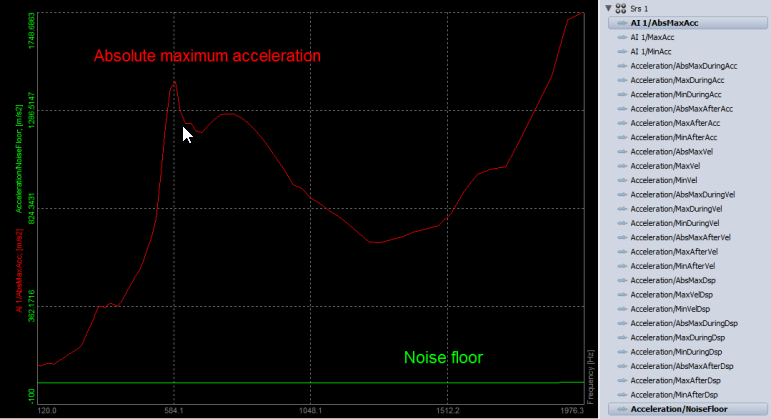# Shock Response Spectrum

The shock response spectrum is a calculated function based on the acceleration time history. It applies an acceleration time history as an excitation to an array of single-degree-of-freedom (SDOF) systems. Each system is assumed to have no mass-loading effect on the base input.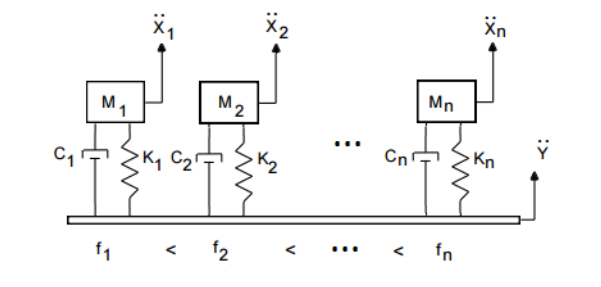𝑌̈ is the base input for each system, and 𝑋̈ is the absolute response of each system to the input (double-dot denotes the acceleration). M is the mass, C is the damping coefficient, and K is the stiffness for each system. The natural frequency for each system is marked with f.

### Input signal

The input signal in the SRS plugin is an impulse shock time signal, usually measured with an accelerometer.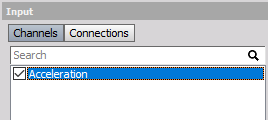###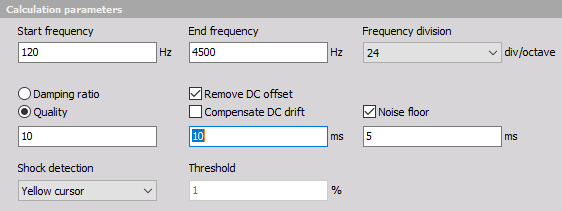Start and end frequency defines the calculation range for the frequency spectrum. The upper limit is set to 1/10 of the MAX sampling rate.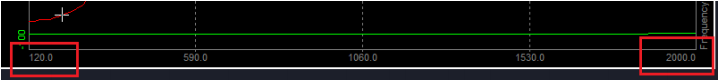Velocity DC-filter removes the DC component of the calculated time signal “InputChannel”/VelocityHistory.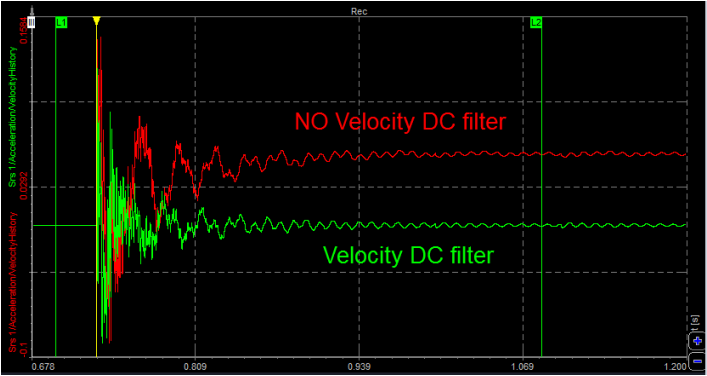Frequency division (octave) defines the resolution of the spectrum in the frequency domain. Higher the number, better the resolution.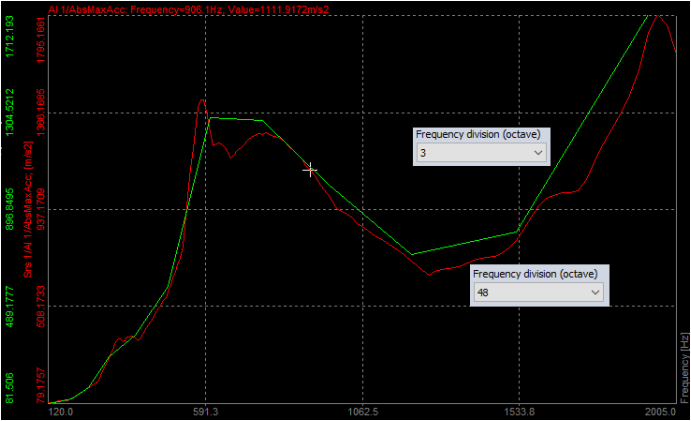Different damping factors produce different SRSs for the same shock waveform. Zero dampings will produce a maximum response and very high damping produces a horizontal line. The standard value for relative damping of 5% results in a Q (quality factor) of 10.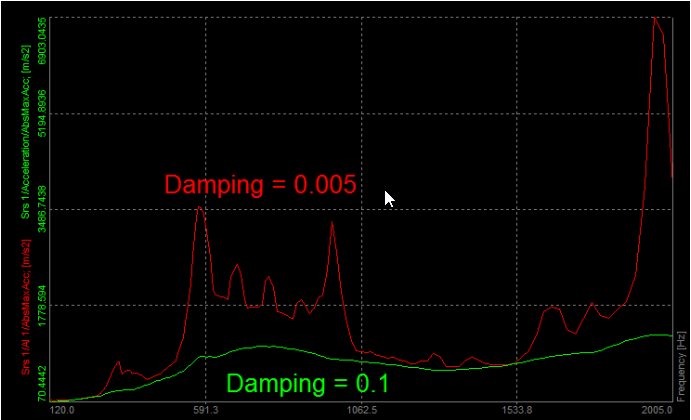Remove DC offset (in milliseconds) removes the DC component of the input time signal. The DC will be calculated as the defined time interval behind the position of the first cursor.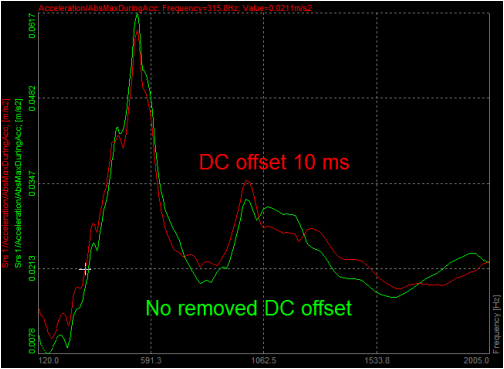Noise floor (in milliseconds) will calculate the noise floor of the input time signal. The noise floor will be calculated as the defined time interval behind the position of the first cursor.

### Results

The results in frequency domain spectrum can be shown as acceleration, velocity, or displacement.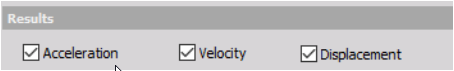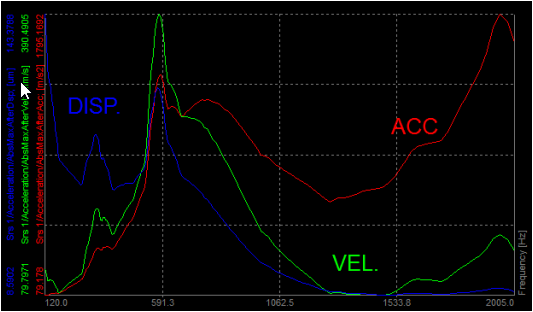### Output channels

As a result, we show absolute maximum, maximum, and minimum of acceleration/velocity/displacement. All of those values are calculated in three different sections: during shock, after shock, and during the whole measurement.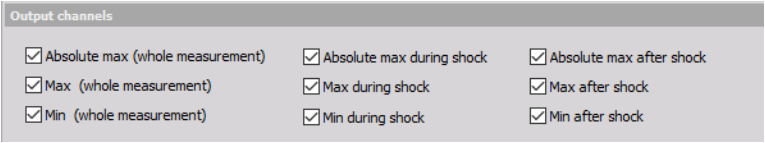• Absolute max (whole measurement) – composite/maximax (the worst-case extrema for both instances). The composite shock response for the absolute maxima is the maximax.
• Max (whole measurement) - composite/maximax (the worst-case extrema for both instances). The composite shock response for the absolute maxima is the maximax.
• Min (whole measurement) - composite/maximax (the worst-case extrema for both instances). The composite shock response for the absolute maxima is the maximax.
• Absolute max during shock - primary (forced response, computation during the shock)
• Max during shock - primary (forced response, computation during the shock)
• Min during shock - primary (forced response, computation during the shock) 7 www.dewesoft.com
• Absolute max after shock - residual (free vibration, computation after the shock)
• Max after shock - residual (free vibration, computation after the shock)
• Min after shock - residual (free vibration, computation after the shock)

### Results

Placing the cursor For the right calculation, we have to place the cursors at the right positions on the recorder. The first cursor (I) has to be placed on the noise floor signal, before the shock. The second cursor (II) has to be placed also on the noise floor but after the shock.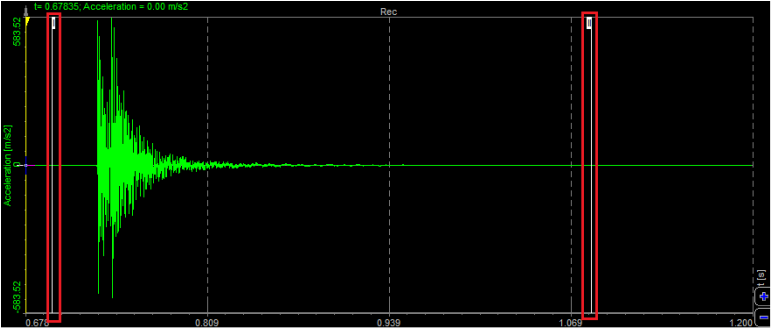After that, the cursors I and II need to be locked with the click on the buttons: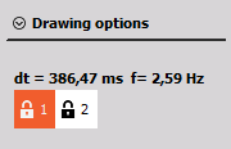After the cursors are locked, they change the color in the recorder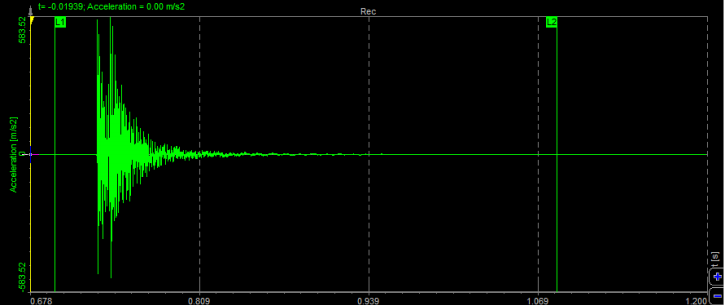The channel cursor (yellow cursor) that indicates our position in the timeline of the datafile has to be placed at the beginning of the shock.

Setup the calculation parameters The next step is to define the calculation parameters (frequency range, damping factor, output channels, …).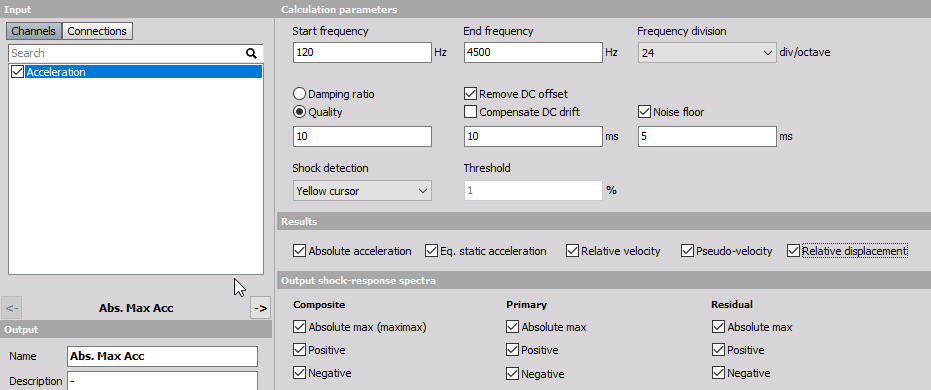After the parameters and cursors are set, we need to Recalculate the data.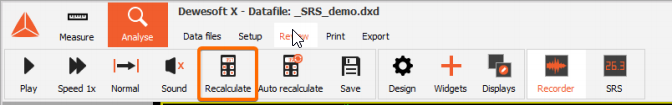Displaying the results The results of the spectrum are calculated in the frequency domain and can be displayed on a 2D graph (“ChannelName/VelocityHistory” is a time channel, displayed on the recorder). Under the channel list, you can select the displayed channels.